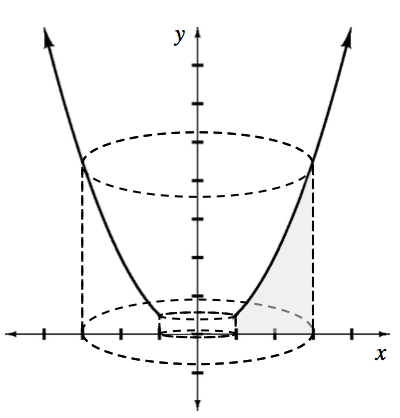### Home > APCALC > Chapter 8 > Lesson 8.1.6 > Problem8-63

8-63.

The diagram at right shows the region bounded by the $x$-axis, $f(x) = 0.5x^2$, $x = 1$, and $x = 3$. The region is revolved about the $y$-axis to create the solid shown with dotted lines. .

1. Describe a method you can use to determine the volume of the solid.

Will you use Washers or Disks? Will the bounds and integrand be written in terms of $x$ or $y$?

2. Set up and evaluate the integrals needed to calculate the volume. (Using washers, the solution will require two integrals.)

The outside of this solid will be a cylinder with radius $3$.

The inside of the solid will have a hole. But what shape is that hole? Is it cylindrical?

$\text{Or is it determined by }f(y) = \sqrt{(2y)}?$

Notice that the bottom of the hole is cylindrical (with radius $1$), while the top of the hole is determined by

$f(y) = \sqrt{(2y)}.$

Consequently, you will need to use the Washer Method twice... both of which are rotated about the $y$-axis.Use the eTool below to help solve the problem.
Click on the link to the right to view the full version of the eTool. Calc 8-63 HW eTool